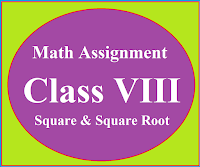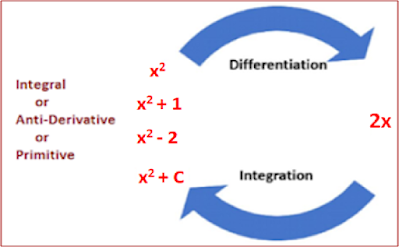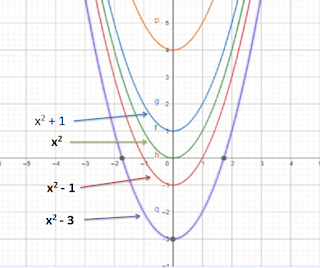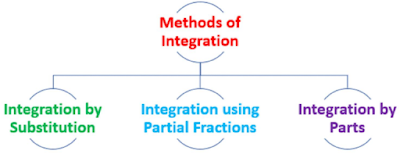### Math Assignment Class VIII | Square & Square RootMath Assignment  Class VIII | Square & Square Root Download or Print free  assignment with answer key  for   Class  8 Squares and  Square Roots.   Important and extra questions that cover all topics of square and square root and is useful and helpful for the students. Math Assignment  Class VIII | Square & Square Root LEVEL -1

### Lesson Plan Math Class 12 (Ch- 7) | Integration

E- LESSON PLAN   SUBJECT MATHEMATICS    CLASS 10+2
Lesson Plan Class 12th Subject Mathematics for Mathematics Teacher. Effective way of Teaching Mathematics. Top planning by the teacher for effective teaching in the class.

 TEACHER'S NAME :   DINESH KUMAR SCHOOL :  RMB DAV CENTENARY PUBLIC SCHOOL, NAWANSHAHR SUBJECT   :   MATHEMATICS CLASS                  :   XII  STANDARDBOARD                 :  CBSE LESSON TOPIC / TITLE   : CHAPTER 07:  INTEGRATION ESTIMATED DURATION: This chapter is divided into 11 modules and is completed in 30 class meetings.

PRE- REQUISITE KNOWLEDGE:-

TEACHING AIDS:-

Green Board, Chalk,  Duster, Charts, smart board, projector, laptop etc.

METHODOLOGY:-

Lecture method, Demonstration and Learning by doing

OBJECTIVES:-
• Integration as inverse process of differentiation.
• Integration of different functions by substitution method.
• Integration of different functions by partial fractions.
• Integration of different functions by by parts (product rule).
• Integration of the Special types of functions.
• Definite integrals as a limit of a sum.
• Fundamental Theorem of Calculus (without proof).
• Basic properties of definite integrals.
• Evaluation of definite integrals.
PROCEDURE :-

Start the session by asking the questions related to the trigonometry, inverse trigonometry and formula's of derivatives. Now introduce the topic Integration step by step as follows.

S. No.

Topic

1

Introduction

First of all teacher will explain the concept that Integration is the reverse process of differentiation by giving some examplesNow teacher will explain the important symbols, terms and phrases related with the integration and their meanings.

2

Types of Integrals: Integrals are of two types Indefinite Integral and Definite Integral.

Explain the relationship(or comparison)  between the differentiation and integration. Also help the students in writing all the

3

Geometrical Meaning of Integration

Now teacher will explain the geometric meaning of integration and some properties of indefinite integrals.4

Different Methods of Integration

Explain different methods of integration like substitution method, Partial Fraction, Integration by parts. Start integration of different functions by substitution method.5

Now teacher will introduce the method of integration by using trigonometric identities, integration of some particular functions.

6

Integration of different functions by partial fractions. Explain the different forms of rational functions and their form in partial fraction and implementation of all these forms in the problems.

7

Now explain the topic Integration of different functions by  parts or product rule in integration.

Also explain the ILATE rule for selecting first function and second function for the students.

## Method of selecting the first function and second function

I...... Inverse Trigonometric Functions

L..... Logarithmic Function

A..... Algebraic Function(Polynomial Function

T......Trigonometric Function

E...... Exponential Function

8

Integration of the functions of the following types.Explain the topic by using related formulas

9

Now introduce the topic Definite integrals. Explain the definite integral as a limit of a sum and explain its geometric meaning to the students.10

Explain both Fundamental Theorem of Calculus (without proof). Theorem 2 : Let f be a continuous function on the closed interval [a , b] and F be the anti derivative of f.Help the students in the implementation of this topic in different problems.

Also explain the method of Integration by substitution method.

11

Now teacher will explain the Basic properties of definite integrals in details and help the students in the implementation of these properties in different problems.

PROPERTIES OF DEFINITE INTEGRAL

⇒ Integration is independent of change of variable.

,  where a < c < b

(OR)

, if f(a-x) = -f(x)

(OR)

, if  f (2a-x) = f(x)

(OR)

,  if f(2a-x) = -f(x)

,  If f(x) is an even function

(OR)
, If f(x) is an odd function

Even function:- If f(-x) = f(x) then, function f(x) is called even function.
Odd Function: If f(-x) = - f(x) then, function f(x) is called odd function.

EXPECTED OUTCOMES:-

After studying this lesson students should understand
• the integration is the inverse process of differentiation.
• Students should knows the substitution method of finding the integration.
• Students should knows the by parts method of finding the integration.
• Students should knows the Partial fraction method of integration.
• integration as the limit of a sum
• Students should knows the properties of definite integral and the evaluation of the definite integrals.
STUDENTS DELIVER ABLES:-
•  Review questions given by the teacher.
• Students should prepare the presentation in groups either on the formula's of  Indefinite Integrals or on Definite Integrals.
• Solve NCERT problems with examples.
EXTENDED LEARNING:-
Students can extend their learning in Mathematics through the RESOURCE CENTRE.  Students can also find many interesting topics on mathematics at cbsemathematics.com

ASSESSMENT TECHNIQUES:-
• Assignment sheet will be given as home work at the end of the topic.
• Separate sheets which will include questions of logical thinking and Higher order thinking skills will be given to the above average students.
• Class Test , Oral Test , worksheet and Assignments. can be made the part of assessment.
• Re-test(s) will be conducted on the basis of the performance of the students in the test.
FEEDBACK:
All those students who performed well will be appreciated positively, and all those who can not perform up to the mark will again be given some important tips, guidance and  positive motivation to go through the topic again and then re-evaluated again. If possible then provision of remedial classes can be made for batter results.

🙏

1.A very good former of lesson plan

2.